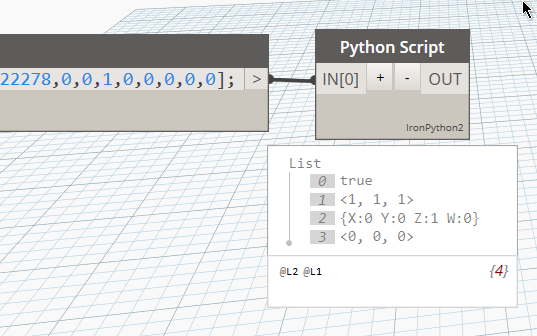# AutoCad Block to Revit family - get block rotation

Hi folks,

I was trying out a workflow where I’m sending blocks from AutoCAD and receiving them in Dynamo in order to place them in Revit as corresponding families. Is there a way to export the Autocad Blocks’ rotation to Speckle? Or could you add this functionality in the future?

This workflow would be handy when working with consultants that use AutoCAD. For example you export your plan in DWG format and send it to an interior consultant. They place their furniture, etc. and send back the DWG. You can then collect all their blocks, match them to some corresponding Revit families in your project, and place those families according to the Cad blocks position and rotation.

2 Likes

Hi @Cillian_Warfield, thanks for reaching out. As far as I am aware, blocks should contain the full position/rotation of the object but I may be mistaken. If not, it is certainly an issue on our side so we’ll definitely look into it and fix it.

It would be super useful if you could share a stream with those blocks to check out the data in Speckle tooThanks for reporting it!!1 Like

I think the rotation is being sent as a `transform` (the property just below `insertionPoint`), I’ll look at the source code and see if I can give you a quick formula to convert between the two1 Like

Alright, so I think I got it! This python script should return:

• if the operation was successful
• scale
• rotation
• translation``````# Load the Python Standard and DesignScript Libraries
import sys
import clr
from System.Numerics import *

v = IN

matrix = Matrix4x4(v,v,v,v,v,v,v,v,v,v,v,v,v,v,v,v)

val = Matrix4x4.Decompose(matrix)

OUT = val
``````
3 Likes

Thanks for the python script! It works in my graph too. However, I’m just not sure how to convert the {X Y Z W} data into radians/degrees. I did some digging in dynamo and figured out that it’s a quaternion(?) but not sure how to proceed.

I tried extracting the Z: data, on the off-chance that that contained the rotation in radians, but that doesn’t seem to be the case.Here’s my extremely hack-y graph for referenceHere’s my stream: Speckle
and I also by the way noticed that my couch blocks are displaying a bit funkily in the 3D viewer.

PS I’m going to log off now, so nobody needs to disturb their evening by answering tonight1 Like

Oops! Sorry, I was in between calls and didn’t notice.
Here’s the code that gives you the angle in degrees, it works on lists as well:

``````import sys
import clr
import math
from System.Numerics import *

def euler_from_quaternion(x, y, z, w):
t0 = +2.0 * (w * x + y * z)
t1 = +1.0 - 2.0 * (x * x + y * y)
roll_x = math.atan2(t0, t1)

t2 = +2.0 * (w * y - z * x)
t2 = +1.0 if t2 > +1.0 else t2
t2 = -1.0 if t2 < -1.0 else t2
pitch_y = math.asin(t2)

t3 = +2.0 * (w * z + x * y)
t4 = +1.0 - 2.0 * (y * y + z * z)
yaw_z = math.atan2(t3, t4)

return roll_x, pitch_y, yaw_z # in radians

#iterate list of transforms
transforms = IN
angles = []

for v in transforms:

matrix = Matrix4x4(v,v,v,v,v,v,v,v,v,v,v,v,v,v,v,v)

decomposed = Matrix4x4.Decompose(matrix)
rotation = decomposed
euler = euler_from_quaternion(rotation.X, rotation.Y, rotation.Z, rotation.W)
angles.append(math.degrees(euler))

OUT = angles
``````

I took the freedom to try with your streamId, it works but will just need some rounding:

P.S.
We’ll look into those weird ellipses being displayed!

2 Likes

That’s awesome, thanks. Super interesting to see the code too. I came across quaternions doing some Unity tutorials a few years ago but alas, I don’t remember much!

1 Like

Oh well, my answer involved a lot of googling!unfortunately c# doesn’t return that automatically!

1 Like

Well your efforts are very much appreciated!
It works like a charm! You can mark this topic/query as solved now (I don’t seem to be able to do that myself).

Also if this workflow is of interest, I can volunteer to create a tutorial based on this for Speckle’s tutorials portal.

4 Likes

Woooh that’d be epic! Yes, pleaseI’ll ping you by email in the next few days so we can coordinate, appreciate it!

2 Likes